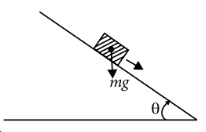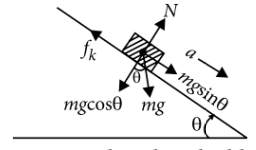Friction on inclined plane of angle more than angle of repose
Question

# A plank with a box on it at one end is gradually raised about the other end. As the angle of inclination with the horizontal reaches 30°, the box starts to slip and slides 4.0m down the plank in 4.0 s. The coefficients of static and kinetic friction between the box and the plank will be, respectivelyDifficult
Solution

## Let  be the coefficients of static and kinetic friction between the box and the plank respectively. When the angle of inclination $\theta$ reaches 30°,  the block just slides,If $\alpha$ is the acceleration produced in the block, then$ma=mg\mathrm{sin}\theta -{f}_{k}$$a=g\left(\mathrm{sin}\theta -{\mu }_{k}\mathrm{cos}\theta \right)$If  s  is the distance travelled by the block in time t,  then But  s = 4.0 m and  t = 4.0 s (given)Substituting this value of $\alpha$ in eqn. (i), we get $\frac{1}{10}=1-\sqrt{3}{\mu }_{k}$${\mu }_{k}=\frac{0.9}{\sqrt{3}}=0.5$

Get Instant Solutions